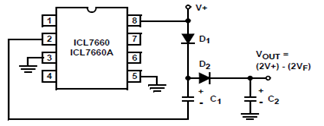# Positive Voltage Doubling Circuit using ICL7660 Voltage Doubler

Positive Voltage Doubling Circuit using ICL7660 Voltage Doubler - The ICL7660 CMOS Voltage Converter can be connected to function as voltage doublersPositive Voltage Doubling Circuit using ICL7660 Voltage Doubler - The ICL7660 CMOS Voltage Converter can be connected to function as voltage doublers in order to generate output voltage.

The schematic herein appears positive voltage doubling circuit using the ICL7660.

In this circuit, the pump inverter switches of the ICL7660 is used to charge C1 to a voltage level of the supply voltage (V+) and the forward voltage drop (Vf) of diode D1.

Voltage on C1 plus the supply voltage is applied through diode D2 to capacitor C2. And the source resistance of the output will depend on the output current.

See ICL7660 application circuit and datasheet for further details.

Tags: Circuit Diagram, Datasheet, voltage doubler, ICL7660, positive voltage,